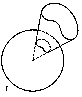# Solid angle

A part of space bounded by all the half-lines going from one point (the vertex) through the points of a closed curve (see Fig. a, Fig. b).Figure: s086060aFigure: s086060b

A particular case of a solid angle is that of a polyhedral angle. One takes as measure of a solid angle the ratio of the surface cut out by it on a sphere centred at the vertex to the squared radius of this sphere. For example, the solid angle containing $1/8$ of the space (an octant) is measured by the number $4\pi R^2/8R^2=\pi/2$. The unit of measurement of solid angles is the steradian.